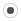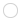## How to use SVD?maxholt
Registered MemberPosts
2
Karma
0

### How to use SVD?

Thu Jul 02, 2009 10:54 pm
I have spent some time testing the example code provided in the two previous forum posts I found on the topic, and I have also tried to adjust the lines of code provided in the tutorial, but I don't quite seem to understand how to use the SVD. Could someone please provide me with a working example code?

I just downloaded Eigen 2.0.3 and tested this bit of code:

Code: Select all
`#include <Eigen/Eigen>using namespace Eigen;int main(){   MatrixXf A = MatrixXf::Random(26,12);       VectorXf b = VectorXf::Random(12);      VectorXf x;      SVD<MatrixXf> svdOfA(A);   std::cout << "..." << std::endl;   svdOfA.solve(b, &x);         const Eigen::Matrix3f U = svdOfA.matrixU();   const Eigen::Matrix3f V = svdOfA.matrixV();   const Eigen::VectorXf S = svdOfA.singularValues();              return 0;}`

It compiles, but after the output "..." the code crashes and the following error message is printed to the console:

Code: Select all
`./Eigen/src/SVD/SVD.h:525: bool Eigen::SVD<MatrixType>::solve(const Eigen::MatrixBase<OtherDerived>&, ResultType*) const [with OtherDerived = Eigen::Matrix<float, 10000, 1, 2, 10000, 1>, ResultType = Eigen::VectorXf, MatrixType = Eigen::Matrix<float, 10000, 10000, 2, 10000, 10000>]: Assertion `b.rows() == rows' failed.Aborted`

Thanks for your help.

Magnusjitseniesen
Registered MemberPosts
204
Karma
2

### Re: How to use SVD?

Fri Jul 03, 2009 10:47 am
It looks like the specified dimensions are not compatible. The matrix A and the vector b should have the same number of rows (that is probably what the mysterious error message is trying to tell you). In your case, the matrix A is 26 x 12 (26 rows, 12 columns), but the vector b has 12 rows. So change
Code: Select all
`VectorXf b = VectorXf::Random(12);`
to
Code: Select all
`VectorXf b = VectorXf::Random(26);`

Disclaimer: I didn't try it myself. I get a mysterious error, probably due to an out-of-date library.jitseniesen
Registered MemberPosts
204
Karma
2

### Re: How to use SVD?

Fri Jul 03, 2009 1:43 pm
There are two more issues:
• You need to specify the dimension of x. This is in disagreement with the documentation, so it should probably be fixed.
• You declare U and V as Matrix3f, which means 3-by-3 matrices, but they need to be bigger. It's easiest to declare them as variable-size.

This leads to the following code, which works on my machine:
Code: Select all
`#include <Eigen/Eigen>using namespace Eigen;int main(){   MatrixXf A = MatrixXf::Random(26,12);   VectorXf b = VectorXf::Random(26);   VectorXf x(12);      SVD<MatrixXf> svdOfA(A);   std::cout << "..." << std::endl;   svdOfA.solve(b, &x);         const Eigen::MatrixXf U = svdOfA.matrixU();   const Eigen::MatrixXf V = svdOfA.matrixV();   const Eigen::VectorXf S = svdOfA.singularValues();             return 0;}`maxholt
Registered MemberPosts
2
Karma
0

### Re: How to use SVD?

Fri Jul 03, 2009 8:12 pm
Thanks!

The size of vector b was a sloppy mistake by me. Also, I concentrated on the solve function so I didn't pay attention to the 3-by-3 matrices at the end. With your suggested size changes, the code now works. This is what it finally looks like (for future reference):

Code: Select all
`#include <iostream>#include <Eigen/Eigen>using namespace Eigen;int main(){   // The following code solves Ax = b, where b is 0, thus making this a homoegeneous linear equation system   // *** LEFT SIDE ***   // Linear equation system is expressed in the form of a matrix of coefficents   // rows-by-cols, m-by-n, 26-by-12, i.e. overdetermined linear equation system   MatrixXf A = MatrixXf::Random(26,12);   std::cout << "Matrix A is:" << std::endl << std::endl << A << std::endl << std::endl;              // Unknowns       VectorXf x(12);       std::cout << "Vector x is:" << std::endl << std::endl << x << std::endl << std::endl;                     // *** RIGHT SIDE ***       // Answer       // Set to zero to solve a homogeneous equation system       VectorXf b = VectorXf::Zero(26);      std::cout << "Vector b is:" << std::endl << std::endl << b << std::endl << std::endl;         SVD<MatrixXf> svdOfA(A);   svdOfA.solve(b, &x);         const Eigen::MatrixXf U = svdOfA.matrixU();   const Eigen::MatrixXf V = svdOfA.matrixV();   const Eigen::VectorXf S = svdOfA.singularValues();      std::cout << "Matrix U is:" << std::endl << std::endl << U << std::endl << std::endl;   std::cout << "Matrix V is:" << std::endl << std::endl << V << std::endl << std::endl;   std::cout << "Matrix S is:" << std::endl << std::endl << S << std::endl << std::endl;   std::cout << "Vector x is:" << std::endl << std::endl << x << std::endl << std::endl;   std::cout << "Vector b is:" << std::endl << std::endl << b << std::endl << std::endl;                    return 0;}`

Slightly OT: I checked the singular values and the values in the last column of V returned by Eigen against those returned by Matlab. Eigen (or Matlab) is accurate down to the fourth decimal which is going to work just nicely for my application.

Thanks.

Magnus

Edit: Typo.

## Who is online

Registered users: Bing [Bot], Google [Bot], jimh, Sogou [Bot], Yahoo [Bot]